Importance: Medium ✭✭
 Author(s): Erdos, Paul Simonovits, Miklos
 Subject: Graph Theory
 Keywords:
 Posted by: fhavet on: March 5th, 2013

Given a finite familyof graphs and an integer, the Turán number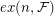ofis the largest integersuch that there exists a graph onvertices withedges which contains no member ofas a subgraph.

Conjecture   For every finite familyof graphs there exists ansuch that.

For the case whenconsists of even cycles, this would mean that (up to constants) the Turán number ofis given by that of the longest cycle in. Verstraëte (see [KO]) conjectured something stronger:

Conjecture   For all integersthere exists a positive c = c(\ell) such that every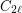-free graphhas a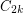-free subgraphwith.

This conjecture was motivated by a result of Györi [G] who showed that every bipartite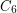-free graphhas a-free subgraph which contains at least half of the edges of. The case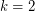was proved in [KO].

## Bibliography

*[ES] P.Erdös and M. Simonovits, Compactness results in extremal graph theory, Combinatorica 2 (1982), 275–288.

[KO] D. Kühn and D. Osthus, 4-cycles in graphs without a given even cycle, J. Graph Theory 48 (2005), 147-156.

[G] E. Györi,-free bipartite graphs and product representation of squares, Discrete Math. 165/166 (1997), 371-375.

* indicates original appearance(s) of problem.